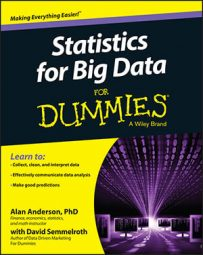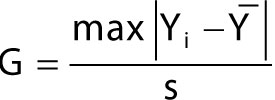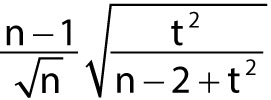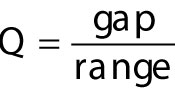##### Statistics for Big Data For DummiesSeveral formal statistical tests that are designed to detect data outliers. Three of these take the form of hypothesis tests. A hypothesis test is a procedure for determining whether a proposition can be rejected based on sample data. Hypothesis tests always involve comparing a test statistic from the data to an appropriate distribution to determine whether a given hypothesis is supported by the data.

## Grubbs' test

With a Grubbs' test, you assume that the dataset being tested for outliers is normally distributed. The null and alternative hypotheses are as follows:

H0: There are no outliers.
H1: There is at least one outlier.

The test statistic is as follows:where

G = The test statistic for the Grubbs' test
Yi = A single element in the dataset being tested
Y = The sample mean
s = The sample standard deviation

The test statistic produces the sample element that is furthest from the sample mean (positive or negative) expressed as standard deviations. For example, if the sample mean is 5, the largest sample element is 11, and the sample standard deviation is 2, then the test statistic would be (11 – 5) / 2 = 6 / 2 = 3 standard deviations away from the mean.

The critical value is as follows:Where

n is the size of the sample drawn from the population.
t is a value drawn from the Student's t-distribution; it has a right tail area equal to the level of significance and n – 2 degrees of freedom (df).

The test can be conducted to determine whether there is an outlier, whether the maximum value is an outlier, whether the minimum value is an outlier, and so on.

For example, the following shows the results of applying Grubbs' test to the S&P 500 returns from 2009–2013. The test is conducted to find a single outlier. Grubbs' test results for one outlier:

Data: SPReturns
G = 3.8509, U = 0.9404, p-value = 0.01177
Alternative hypothesis: Lowest value –0.0253283545257448 is an outlier

With a level of significance equal to 0.05, and a p-value of 0.01177, the p-value is below the level of significance. Therefore, the null hypothesis of no outliers is rejected. Furthermore, the test indicates that the minimum value in the dataset is an outlier.

## Chi-square test

You can test for outliers with the chi-square distribution. The null and alternative hypotheses are as follows:

H0: There are no outliers.
H1: There is at least one outlier.

The test statistic is based on the differences between the actual members of a dataset and the corresponding members of an assumed probability distribution, such as the normal.

For example, the following shows the results of applying the chi-square test to the S&P 500 returns from 2009–2013:

Chi-square test for outlier
Data: SPReturns
X-squared = 14.8292, p-value = 0.01177
Alternative hypothesis: Lowest value –0.0253283545257448 is an outlier

With a level of significance equal to 0.05, and a p-value of 0.01177, the p-value is below the level of significance. Therefore, the null hypothesis of no outliers is rejected. Furthermore, the test indicates that the minimum value in the dataset is an outlier.

## Dixon's Q test

With Dixon's Q test, you assume the dataset being tested for outliers is normally distributed. The null and alternative hypotheses are as follows:

H0: There are no outliers.
H1: There is at least one outlier.

The test statistic is as follows:Gap refers to the absolute value of the difference between an outlier and the next closest value in the dataset. Range refers to the difference between the largest value in the dataset and the smallest value in the dataset.

One of the drawbacks to Dixon's Q test is that you can apply it only to a sample containing between 3 and 30 observations.

The following shows the results of applying Dixon's Q test to the S&P 500 returns during the first 30 trading days of 2009:

Dixon test for outliers
Data: SPR
Q = 0.4359, p-value = 0.03185
Alternative hypothesis: Lowest value –0.0116057775514049 is an outlier

With a level of significance equal to 0.05, and a p-value of 0.03185, the p-value is below the level of significance. Therefore, the null hypothesis of no outliers is rejected. Furthermore, the test indicates that the minimum value in the dataset is an outlier.